# The Maximal Flow Problem[Page 286]

In the shortest route problem we determined the shortest truck route from the origin (Los Angeles) to six destinations. In the minimal spanning tree problem we found the shortest connected network for television cable. In neither of these problems was the capacity of a branch limited to a specific number of items. However, there are network problems in which the branches of the network have limited flow capacities . The objective of these networks is to maximize the total amount of flow from an origin to a destination. These problems are referred to as maximal flow problems .

The maximal flow problem is to maximize the amount of flow of items from an origin to a destination.

Maximal flow problems can involve the flow of water, gas, or oil through a network of pipelines; the flow of forms through a paper processing system (such as a government agency); the flow of traffic through a road network; or the flow of products through a production line system. In each of these examples, the branches of the network have limited and often different flow capacities. Given these conditions, the decision maker wants to determine the maximum flow that can be obtained through the system.

An example of a maximal flow problem is illustrated by the network of a railway system between Omaha and St. Louis shown in Figure 7.18. The Scott Tractor Company ships tractor parts from Omaha to St. Louis by railroad. However, a contract limits the number of railroad cars the company can secure on each branch during a week.

##### Figure 7.18. Network of railway system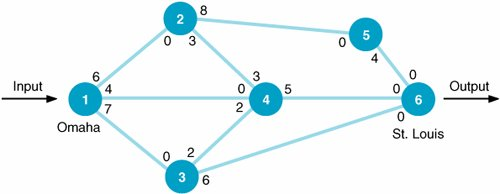Given these limiting conditions, the company wants to know the maximum number of railroad cars containing tractor parts that can be shipped from Omaha to St. Louis during a week. The number of railroad cars available to the tractor company on each rail branch is indicated by the number on the branch to the immediate right of each node (which represents a rail junction). For example, six cars are available from node 1 (Omaha) to node 2, eight cars are available from node 2 to node 5, five cars are available from node 4 to node 6 (St. Louis), and so forth. The number on each branch to the immediate left of each node is the number of cars available for shipping in the opposite direction. For example, no cars are available from node 2 to node 1. The branch from node 1 to node 2 is referred to as a directed branch because flow is possible in only one direction (from node 1 to node 2, but not from 2 to 1). Notice that flow is possible in both directions on the branches between nodes 2 and 4 and nodes 3 and 4. These are referred to as undirected branches .

For a directed branch, flow is possible in only one direction .

#### The Maximal Flow Solution Approach

The first step in determining the maximum possible flow of railroad cars through the rail system is to choose any path arbitrarily from origin to destination and ship as much as possible on that path. In Figure 7.19 we will arbitrarily select the path 1256. The maximum number of railroad cars that can be sent through this route is four. We are limited to four cars because that is the maximum amount available on the branch between nodes 5 and 6. This path is shown in Figure 7.19.

[Page 287]
##### Figure 7.19. Maximal flow for path 1256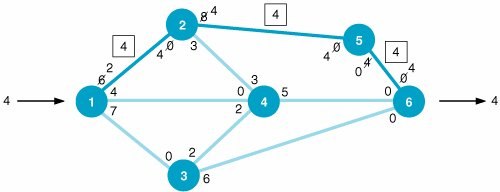Arbitrarily choose any path through the network from origin to destination and ship as much as possible .

## Time Out: For E. W. Dijkstra, L. R. Ford, Jr., and D. R. Fulkerson

In 1959 E. W. Dijkstra of the Netherlands proposed the solution procedures (shown in this chapter) for both the minimal spanning tree problem and the shortest route problem. Earlier, in 1955, L. R. Ford, Jr., and D. R. Fulkerson (colleagues of George Dantzig's) of the RAND Corporation introduced the procedure for solving the maximal flow network problem, which evolved from the study of transportation problems. The original problem analyzed was of a rail network connecting cities in which each rail link had capacity limitations.

Notice that the remaining capacities of the branches from node 1 to node 2 and from node 2 to node 5 are two and four cars, respectively, and that no cars are available from node 5 to node 6. These values were computed by subtracting the flow of four cars from the number available. The actual flow of four cars along each branch is shown enclosed in a box. Notice that the present input of four cars into node 1 and output of four cars from node 6 are also designated.

Recompute branch flow in both directions .

The final adjustment on this path is to add the designated flow of four cars to the values at the immediate left of each node on our path, 1256. These are the flows in the opposite direction. Thus, the value 4 is added to the zeros at nodes 2, 5, and 6. It may seem incongruous to designate flow in a direction that is not possible; however, it is the means used in this solution approach to compute the net flow along a branch. (If, for example, a later iteration showed a flow of one car from node 5 to node 2, then the net flow in the correct direction would be computed by subtracting this flow of one in the wrong direction from the previous flow of four in the correct direction. The result would be a net flow of three in the correct direction.)

We have now completed one iteration of the solution process and must repeat the preceding steps. Again, we arbitrarily select a path. This time we will select path 146, as shown in Figure 7.20. The maximum flow along this path is four cars, which is subtracted at each of the nodes. This increases the total flow through the network to eight cars (because the flow of four along path 146 is added to the flow previously determined in Figure 7.19).

##### (This item is displayed on page 288 in the print version)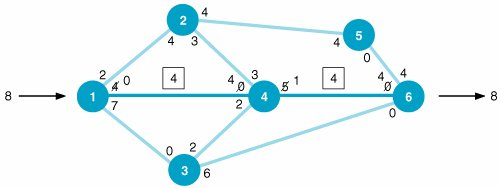Select other feasible paths arbitrarily and determine maximum flow along the paths until flow is no longer possible.

As a final step, the flow of four cars is added to the flow along the path in the opposite direction at nodes 4 and 6.

[Page 288]

Now we arbitrarily select another path. This time we will choose path 136, with a maximum possible flow of six cars. This flow of six is subtracted from the branches along path 136 and added to the branches in the opposite direction, as shown in Figure 7.21. The flow of 6 for this path is added to the previous flow of 8, which results in a total flow of 14 railroad cars.

[Page 289]
##### (This item is displayed on page 288 in the print version)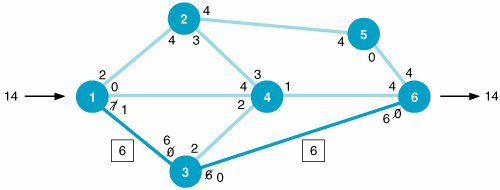## (This item is displayed on page 288 in the print version)

Yellow Freight System is one of the largest motor freight carriers in the United States, handling more than 15 million shipments over a network of 380 terminals annually. Revenues in 1997 exceeded \$2.5 billion. Yellow Freight primarily concentrates on the less-than -truckload (LTL) market. LTL shipments are typically less than 10,000 pounds , and because tractor-trailer trucks can normally accommodate about 45,000 pounds , LTL shipments are consolidated to form economical, full-truck shipments. This required an extensive network of terminals where the individual LTL shipments are consolidated and loaded (i.e., break bulking) for individual delivery in the area. In order to manage its increasingly complex terminal network system, Yellow Freight developed a network modeling approach called SYSNET to improve customer service and reliability, and, secondarily, to improve productivity and lower costs. SYSNET is used for monthly load planning that governs how shipments are consolidated and handled throughout the network and for long-range planning, including the location and size requirements of the company's facilities.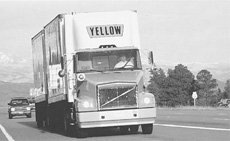Source: J. W. Braklow, et al., "Interactive Optimization Improves Service and Performance for Yellow Freight System," Interfaces 22, no. 1 (JanuaryFebruary 1992): 14772.

Notice that at this point the number of paths we can take is restricted. For example, we cannot take the branch from node 3 to node 6 because zero flow capacity is available. Likewise, no path that includes the branch from node 1 to node 4 is possible.

The available flow capacity along the path 1346 is one car, as shown in Figure 7.22. This increases the total flow from 14 cars to 15 cars. The resulting network is shown in Figure 7.23. Close observation of the network in Figure 7.23 shows that there are no more paths with available flow capacity. All paths out of nodes 3, 4, and 5 show zero available capacity, which prohibits any further paths through the network.

##### Figure 7.22. Maximal flow for path 1346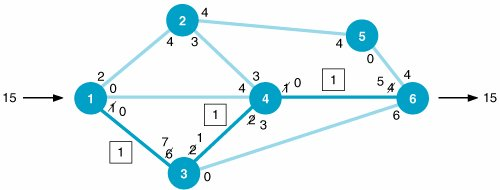##### Figure 7.23. Maximal flow for railway network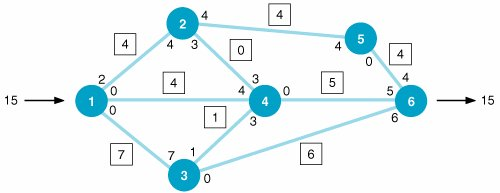This completes the maximal flow solution for our example problem. The maximum flow is 15 railroad cars. The flows that will occur along each branch appear in boxes in Figure 7.23.

In summary, the steps of the maximal flow solution method are as follows :

1. Arbitrarily select any path in the network from origin to destination.

2. Adjust the capacities at each node by subtracting the maximal flow for the path selected in step 1.

3. Add the maximal flow along the path in the opposite direction at each node.

4. Repeat steps 1, 2, and 3 until there are no more paths with available flow capacity.

Steps of the maximal flow solution method .

#### Computer Solution of the Maximal Flow Problem with QM for Windows

The program input and maximal flow solution for the Scott Tractor Company example using QM for Windows is shown in Exhibit 7.7. (Note that this problem has multiple optimal solutions, and the branch flows are slightly different for this solution.)

[Page 290]
##### Exhibit 7.7.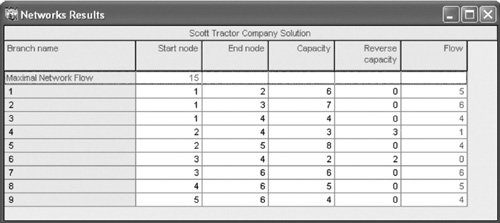#### Computer Solution of the Maximal Flow Problem with Excel

The maximal flow problem can also be solved with Excel, much the same way as we solved the shortest route problem, by formulating it as an integer linear programming model and solving it by using the "Solver" option from the "Tools" menu.

The decision variables represent the flow along each branch, as follows:

x ij = flow along branch ij and integer

To reduce the size and complexity of the model formulation, we will also eliminate flow along a branch in the opposite direction (e.g., flow from 4 to 2 is zero).

Before proceeding with the model formulation and developing the objective function, we must alter the network slightly to be able to solve it as a linear programming problem. We will create a new branch from node 6 back to node 1, x 61 . The flow along this branch from the end of the network back to the start corresponds to the maximum amount that can be shipped from node 6 to node 1 and then back through the network to node 6. In effect, we are creating a continual flow through the network so that the most that goes through and comes out at node 6 is the most that can go through the network beginning at node 1. As a result, the objective is to maximize the amount that flows from node 6 back to node 1:

maximize Z = x 61

The constraints follow the same premise as the shortest route problem; that is, whatever flows into a node must flow out. Thus, at node 1 we have the following constraint, showing that the amount flowing from 61 must flow out through branches 12, 13, and 14:

x 61 = x 12 + x 13 + x 14

This constraint can be rewritten as

x 61 x 12 x 13 x 14 = 0

Similarly, the constraint at node 2 is written as

x 12 x 24 x 25 = 0

We must also develop a set of constraints reflecting the capacities along each branch, as follows:

 x 12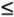6 x 342 x 137 x 366 x 144 x 465 x 243 x 564 x 258 x 6117

[Page 291]

The capacity for x 61 can be any relatively large number (compared to the other branch capacities); we set it at the sum of the capacities on the branches leaving node 1.

The complete linear programming model for our Scott Tractor Company example is summarized as follows: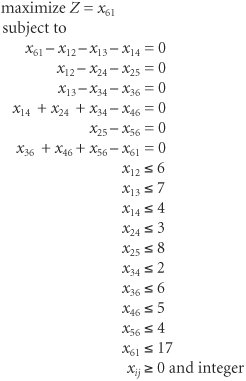The Excel spreadsheet set up to solve this integer linear programming model for the Scott Tractor Company example is shown in Exhibit 7.8. The decision variables, x ij , are represented by cells C6:C15 . Cells D6:D15 include the branch capacities. The objective function, which is to maximize the flow along branch 61, is included in cell C16. The model constraints reflecting the flow through each node are included in the box on the right side of the spreadsheet. For example, cell G6 contains the constraint formula at node 1, =C15C6C7C8 , and cell G7 contains the constraint formula for node 2, =C6C9C10 . The constraints for the branch capacities are obtained by adding the formula C6:C15D6:D15 in Solver.

##### Exhibit 7.8.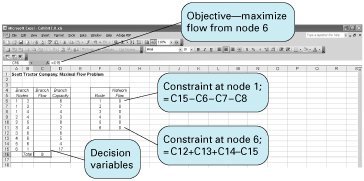[Page 292]

Exhibit 7.9 shows the Solver Parameters screen for this model.

##### Exhibit 7.9.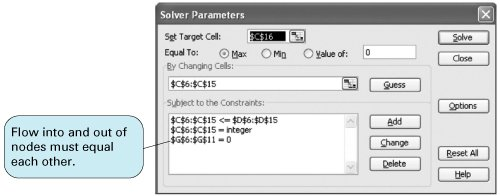The Excel solution is shown in Exhibit 7.10. Notice that the flows along each branch are shown in cells C6:C15 and the total network flow is 15 in cell C16.

##### Exhibit 7.10.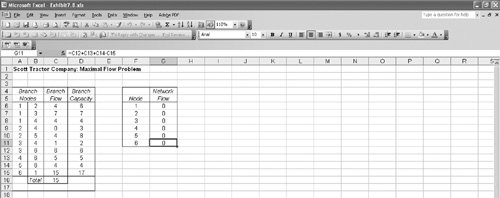Introduction to Management Science (10th Edition)
ISBN: 0136064361
EAN: 2147483647
Year: 2006
Pages: 358

Similar book on Amazon

flylib.com © 2008-2017.
If you may any questions please contact us: flylib@qtcs.net